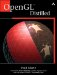# Section 2.1. OpenGL Primitives

### 2.1. OpenGL Primitives

In OpenGL, applications render primitives by specifying a primitive type and a sequence of vertices with associated data. The primitive type determines how OpenGL interprets and renders the sequence of vertices.

#### 2.1.1. Primitive Types

OpenGL provides ten different primitive types for drawing points, lines, and polygons, as shown in Figure 2-1.

##### Figure 2-1. OpenGL primitive types.OpenGL interprets the vertices and renders each primitive using the following rules:

• GL_POINTS Use this primitive type to render mathematical points. OpenGL renders a point for each vertex specified.

• GL_LINES Use this primitive to draw unconnected line segments. OpenGL draws a line segment for each group of two vertices. If the application specifies n vertices, OpenGL renders n/2 line segments. If n is odd, OpenGL ignores the final vertex.

• GL_LINE_STRIP Use this primitive to draw a sequence of connected line segments. OpenGL renders a line segment between the first and second vertices, between the second and third, between the third and fourth, and so on. If the application specifies n vertices, OpenGL renders n1 line segments.

• GL_LINE_LOOP Use this primitive to close a line strip. OpenGL renders this primitive like a GL_LINE_STRIP with the addition of a closing line segment between the final and first vertices.

• GL_TRIANGLES Use this primitive to draw individual triangles. OpenGL renders a triangle for each group of three vertices. If your application specifies n vertices, OpenGL renders n/3 triangles. If n isn't a multiple of 3, OpenGL ignores the excess vertices.

• GL_TRIANGLE_STRIP Use this primitive to draw a sequence of triangles that share edges. OpenGL renders a triangle using the first, second, and third vertices, and then another using the second, third, and fourth vertices, and so on. If the application specifies n vertices, OpenGL renders n2 connected triangles. If n is less than 3, OpenGL renders nothing.

• GL_TRIANGLE_FAN Use this primitive to draw a fan of triangles that share edges and also share a vertex. Each triangle shares the first vertex specified. If the application specifies a sequence of vertices v, OpenGL renders a triangle using v0, v1, and v2; another triangle using v0, v2, and v3; another triangle using v0, v3, and v4; and so on. If the application specifies n vertices, OpenGL renders n2 connected triangles. If n is less than 3, OpenGL renders nothing.

• GL_QUADS Use this primitive to draw individual convex quadrilaterals. OpenGL renders a quadrilateral for each group of four vertices. If the application specifies n vertices, OpenGL renders n/4 quadrilaterals. If n isn't a multiple of 4, OpenGL ignores the excess vertices.

• GL_QUAD_STRIP Use this primitive to draw a sequence of quadrilaterals that share edges. If the application specifies a sequence of vertices v, OpenGL renders a quadrilateral using v0, v1, v3, and v2; another quadrilateral using v2, v3, v5, and v4; and so on. If the application specifies n vertices, OpenGL renders (n-2)/2 quadrilaterals. If n is less than 4, OpenGL renders nothing.

• GL_POLYGON Use GL_POLYGON to draw a single filled convex n-gon primitive. OpenGL renders an n-sided polygon, where n is the number of vertices specified by the application. If n is less than 3, OpenGL renders nothing.

For GL_QUADS, GL_QUAD_STRIP, and GL_POLYGON, all primitives must be both planar and convex. Otherwise, OpenGL behavior is undefined. The GLU library supports polygon tessellation, which allows applications to render filled primitives that are nonconvex or self-intersecting, or that contain holes. See the "gluTess" set of functions in OpenGL® Reference Manual for more information.

#### 2.1.2. Vertex Sharing

Note that GL_LINE_STRIP, GL_LINE_LOOP, GL_TRIANGLE_STRIP, GL_TRIANGLE_FAN, and GL_QUAD_STRIP all share vertices among their component line segments, triangles, and quadrilaterals. In general, you should use these primitives when possible and practical to reduce redundant per-vertex computation.

You could render a two-quadrilateral GL_QUAD_STRIP primitive by using GL_QUADS, for example. Rendered as a GL_QUAD_STRIP, your application would need to send only six unique vertices. The GL_QUADS version of this primitive, however, would require eight vertices, two of which are redundant. Passing identical vertices to OpenGL increases the number of per-vertex operations and could create a performance bottleneck in the rendering pipeline.OpenGL Distilled
ISBN: 0321336798
EAN: 2147483647
Year: 2007
Pages: 123
Authors: Paul Martz